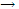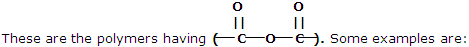What is Flash Photolysis Reactions. Explain with examples.

An example illustrates the type of mechanism that can be written to explain the development of flash photolysis reactions.

Often, as the reactions in the ozone layer of the earth's atmosphere, we are interested in the kinetic behavior of species that are not available as on the shelf chemicals. The rate constants of reactions involving such species as H atoms, O atoms, and OH and HO2 radicals must be known if the dynamics of complex reaction mixtures are to be understood. Rate constant data can sometimes be obtained by generating such species by a high intensity short duration flash of light and then following their subsequent reaction. This approach is known as flash photolysis.

A typical flash used in such studies can generate about 1 mol of produces in the several microsecond duration of the flash. The amount of one or more of the products formed directly or indirectly, is usually determined by measuring the absorption of light at a suitable wavelength. As an illustration of this technique, studies of the formation and reaction of the perhydroxyl radical,H2O are described.

The two reactions are:

H2O = hvOH + H

OH + H2H2O + H

The formation of HO2 radicals by this flash photolysis route and studies of the kinetics of subsequent reactions has been reported. The reaction system contained small amounts of H2Oand O2 and enough H2 to produce a total pressure of 1 atm. The formation of H atoms from the photolysis at water and their attachment to O2 to form HO2 occurred rapidly. Within about 10μs the intermediates, H and OH, were largely consumed. The kinetics of the subsequent reactions ofH2O radicals could then be studied.

The optional transmission at 210 nm, where HO2 absorbs, can be used to follow the decrease of this species. If the reaction that removes HO2 is second order in HO2, the H2O concentration will vary with the time according to:

1/c = 1/ c0 + kt

That the reaction is indeed order is confirmed by the linear relation for 1/ {log (I0 /i)}, which is proportional to 1/c, versus t, as shown in this order and the net product of the reaction, H2O2, suggest that the decay of H2O occurs by the reaction:

HO2 + HO2H2O+ O2

The reaction following the initial photolysis process can be changed by changing the reagents in the reaction system.

The principle reaction sequent system then is:

OH + HO2H2O + O2

Principal reactions used for the simulation curves:

 Reaction Rate constant OH + H2H2O + H 4 × 104 L mol-1 s-1 H + O2 H2HO2 + H2 2 × 1010 L2 mol-2 s-1 H + O2 + ArHO2 + Ar 6 × 109 L2 mol-2 s-1 H + O2 + H2OHO2 + H2O 1.4 × 1011 L2 mol-2 s-1 HO2 + HO2H2O2 6 × 109 L mol-1 s-1 OH + HO2H2O + O3 1.2 × 1011 L mol-1 s-1

#### Related Questions in Chemistry

• ##### Q :Osmotic Pressure The O.P. (Osmotic

The O.P. (Osmotic Pressure) of equimolar solution of Urea, BaCl2 and AlCl3, will be in the order:(a) AlCl3 > BaCl2 > Urea  (b) BaCl2 > AlCl3 > Urea  (c) Urea > BaCl2<

• ##### Q :HCl is an acid or a base Illustrate is

Illustrate is HCl an acid or a base ?

• ##### Q :Calculating Formulae Superphosphate has

Superphosphate has the formula CaH4(PO4)2 H2O, what is the calculation to get the percentage of Phosphorus, I need to show the calculation. I know it is 30.9737622 u in weight and 2 atoms of the formula, but not sure how to work the calculation backwards.

• ##### Q :Problem on reversible process a. For a

a. For a reversible process involving ideal gases in a closed system, Illustrate thatΔS = Cv ln(T2/T1) for a constant volume process ΔS = Cp ln(T2/T1) for a constant pressu

• ##### Q :Problem on vapour pressure Choose the

Choose the right answer from following. If P and P are the vapour pressure of a solvent and its solution respectively N1 and N2 and are the mole fractions of the solvent and solute respectively, then correct relation is: (a) P= PoN1 (b) P= Po N2 (c)P0= N2 (d)

• ##### Q :What are condensation polymers? Give

These types of polymers are formed as a result of condensation reaction between monomer units. Some common examples are being discussed here: 1. Polyesters##### Q :Haloalkanes define primary secondary

define primary secondary and tertiary alkyl halides with examples

• ##### Q :Neutralization of benzoic acid Choose

Choose the right answer from following. How many grams of NaOH will be required to neutralize 12.2 grams of benzoic acid : (a) 40gms (b) 4gms (c)16gms (d)12.2gms

• ##### Q :Define thermal energy The thermal part

The thermal part of the internal energy and the enthalpy of an ideal gas can be given a molecular level explanation. All the earlier development of internal energy and enthalpy has been "thermodynamic". We have made no use o

• ##### Q :Concentration of an aqueous solution

Give me answer of this question. The concentration of an aqueous solution of 0.01M CH3OH solution is very nearly equal to which of the following : (a) 0.01%CH3OH (b) 0.1%CH3OH (c) xCH3OH= 0.01 (d) 0.99MH2O (